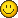# Graphics distortion

## Recommended Posts

Im having a problem while playing KotOR II. Here's the deal. I installed it, updated the game and tried to play. It ran fine until I got to the Mining Colony (forgot name). Now, the graphics get distorted at random time. It's like the polygons in the models get stretch out and end in a sharp point, and it can range from ignorable to unplayable.

I tried the Disable Vertex Buffer Objects=1 trick to see if it help alleviate the problem, and it did. Unfortunaely it did so at the cost of fps, to the point where the game runs at a crawl.

Any ideas on what the problem could be? Im lost. Here my swinfo.txt if it helps.

[sWKotOR]

ReportDateTime=5/17/2005 3:24:07 AM

SysInfoVersion=v1.00.60

GameExists=1

GameVersion=v2.10.427

GameInstallLocation=C:\Program Files\LucasArts\SWKotOR2\

[OS]

Name=WinXP

Version=Windows XP v5.1 build 2600 Service Pack 2

Service Pack=Service Pack 2

Status=Pass

[swapFiles]

C:\pagefile.sys=1536

[CPU]

CPUCount=1

CPUSpeed=2157

CPUFamily=6

CPUModel=8

CPUStepping=1

CPUVendor=AMD

CPUName=AMD Athlon XP 2700+

Status=Pass

[Memory]

RAM=1024

Status=Pass

[Disk Free Space]

C: (NTFS), UNICODE=24.67GB

Status=Pass

[CD-ROMs]

DriveLetters=D:\

Drives=PIONEER DVD-ROM DVD-116

[Video]

Video Memory=256

Desktop Resolution=1280x1024x16 @ 60Hz

DirectX=DirectX 9.0c (4.09.00.0904)

OpenGL Version=2.0.5014 WinXP Release

OpenGL Vendor=ATI Technologies Inc.

ATI Driver=6.14.10.6525

Vid Card Status=Pass

Vid Card Driver Status=Pass

GL Status=Pass

DX Status=Pass

[Audio]

Sound Card Name=Creative SB Extigy

Status=Pass

;Game Options from swkotor2.ini

[Display Options]

FullScreen=1

Disable Movies=0

Disable Intro Movies=0

Sort Modules=1

Width=640

Height=480

BitsPerPixels=32

RefreshRate=60

[sound Options]

Music Volume=85

Voiceover Volume=85

Sound Effects Volume=85

Movie Volume=85

Number 3D Voices=32

Number 2D Voices=32

2D3D Bias=1.00

EAX=0

Sound Init=0

Disable Sound=0

Force Software=1

[Graphics Options]

Grass=0

EnableHardwareMouse=1

FullScreen=1

RefreshRate=60

Width=1024

Height=768

Emitters=1

Anisotropy=16

Frame Buffer=1

Brightness=57

V-Sync=1

Texture Quality=2

Anti Aliasing=6

[Game Options]

Enable Tooltips=1

Keyboard Camera Deceleration=2000.000000

Keyboard Camera Acceleration=500.000000

Keyboard Camera DPS=200.000000

TextureVariation=0

BodyVariation=0

AppearanceType=0

PCGender=0

GameCompleted=0

CurSithLord=0

Hide InGame GUI=0

Use Small Fonts=0

Hide Unequippable=0

Tutorial Popups=1

Subtitles=1

Mini Map=1

Floating Numbers=1

Status Summary=1

Enable Mouse Teleporting To Buttons=1

Mouse Sensitivity=44

Difficulty Level=1

Auto Level Up NPCs=0

Mouse Look=0

AutoSave=1

EnableScreenShot=0

Reverse Mouse Buttons=0

Reverse Ingame YAxis=0

Reverse Minigame YAxis=0

Combat Movement=1

UnlockedPlanetSongs=2

TooltipDelay Sec=1

Disable Movies=0

[config]

firstrun=0

[Keymapping]

Action286B=8

Action286A=7

Action285B=10

Action285A=9

Action284B=54

Action284A=51

Action283B=54

Action283A=51

Action282B=69

Action282A=73

Action281B=53

Action281A=76

Action280B=69

Action280A=73

Action268=52

Action265=58

Action264=57

Action263=43

Action262=85

Action261=84

Action260=83

Action259=82

Action258=81

Action257=80

Action256=79

Action255=78

Action254=77

Action253=31

Action245=75

Action244=55

Action243=67

Action242=74

Action241=87

Action240=56

Action239=68

Action238=82

Action236=83

Action234=81

Action232=80

Action230=79

Action228=78

Action226=77

Action225=70

Action224=90

Action223=31

Action222=25

Action221=24

Action220=29

Action219=28

Action218=42

Action217=87

Action216=65

Action215=63

Action214=60

Action213=62

Action212=61

Action211=66

Action210=59

Action209=71

Action208=89

Action207=72

Action206=30

Action205=55

Action204=67

[Autopause Options]

End Of Combat Round=0

Mine Sighted=0

Enemy Sighted=1

Party Killed=1

New Target Selected=1

[Movies Shown]

Movie10=0

Movie 9=0

Movie 8=0

Movie 7=0

Movie 6=0

Movie 5=0

Movie 4=0

Movie 3=0

Movie 2=0

Movie 1=0

Movie 0=0

##### Share on other sites

I have a similar system and had similar problems before adding the Vertex Buffers line to .ini. I even had a surreal experience where Exile was walking far above the ground on Korriban. The change to the .ini file didn't noticably impact my frame rate, but the only difference I can see in our Graphics options is that I have Anti-aliasing set lower (4) and Anisotropy (. Oh, and I do have grass and shadows on, with a refresh of 85. I don't know if that's much help.##### Share on other sites

wait a minute. using disable vertex buffer objects=1 command line reduces fps? I didn't even notice. Maybe that's why it's unplayable at 8x anti-aliasing... Even if I could get higher fps, without this command line I would have some annoying issues on Dantooine.

##### Share on other sites

I probably should have used the correct swsinfo.txt. That one was from when I dicked around with the graphics options to see if it would help. It was sluggish on 800x600, all options off.

It was the catalyst 5.4 drivers that were the cause, for whatever reason. Rolled back through them all and stuck with the 5.1's .Full graphics options now, with no distortion.